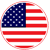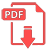# Home | Mathematics | Geometry(Planimetry)

A quadrilateral, in general, has sides of different lengths and angles of different measures. However, squares, rectangles, etc. are special types of quadrilaterals with some of their sides and angles being equal. This is the reason that the area of quadrilateral depends on which type of quadrilateral it is. In this article, we will discuss the special types of quadrilaterals and their basic properties.

There are six basic types of quadrilaterals. They are:

Trapezium
Parallelogram
Rectangle
Rhombus
Square
KiteEnglish